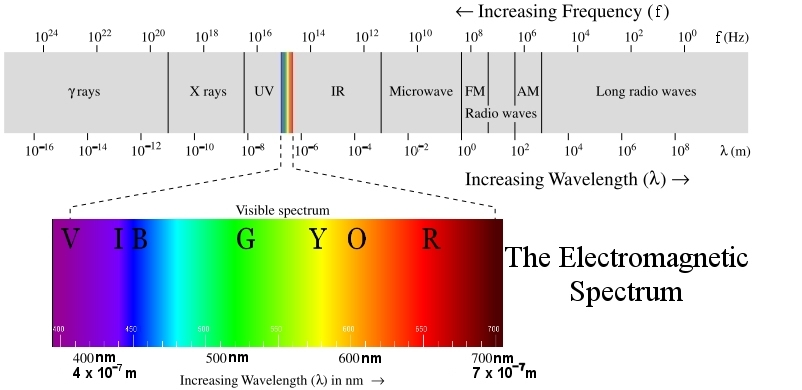λ stands for wavelength in meters (m)
f stands for frequency in hertz (Hz)

A short wavelength has a large frequency and a high energy per photon:
small λ —> large f —> large E per photon

A long wavelength has a small frequency and a small energy per photon:
large λ —> small f —> small E per photon
Here is a page about mnemonics to help you remember the order of the colors of the rainbow.
 Constants of Nature Speed of Light c 3.00 × 108 m/s Planck’s Constant h 4.136 × 10–15 eV· s 6.626 × 10–34 J· s
Important Formulas c = λf
(relates frequency and wavelength of light)
E = hf
(relates energy of one photon and frequency of light)
Important Conversions
micrometers (μm): 1 m = 1 × 106 μm
nanometers (nm): 1 m = 1 × 109 nm
gigahertz (GHz): 1 GHz = 1 × 109 Hz
megahertz (MHz): 1 MHz = 1 × 106 Hz
kilohertz (kHz): 1 kHz = 1,000 Hz
electron volts (eV) and joules (J):
1 eV = 1.602 × 10–19 J
This page is a reference document meant to be used with this Activity: Light.
Last updated: Jun 01, 2022       Home